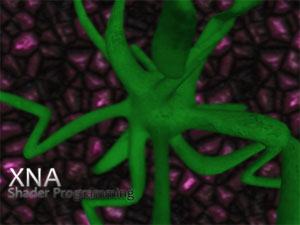## 景深（Depth of Field）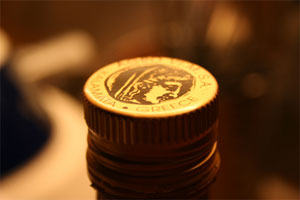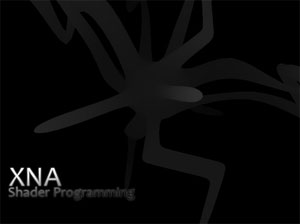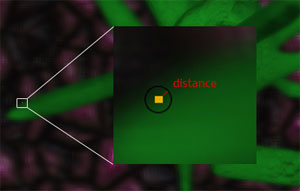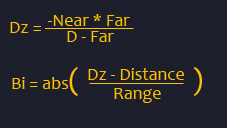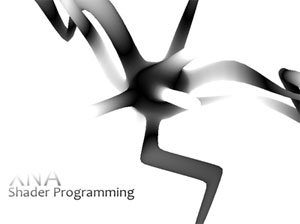// 模糊程度( 即提取当前像素的周围像素的范围)
float BlurDistance = 0.003f;
// This will use the texture bound to the object( like from the sprite batch ).
sampler ColorMapSampler : register(s0);

float4 PixelShader(float2 Tex: TEXCOORD0) : COLOR
{
float4 Color;

// 使用修改过的纹理坐标从ColorMapSampler采样颜色，并将颜色叠加。
Color  = tex2D( ColorMapSampler, float2(Tex.x+BlurDistance, Tex.y+BlurDistance));
Color += tex2D( ColorMapSampler, float2(Tex.x-BlurDistance, Tex.y-BlurDistance));
Color += tex2D( ColorMapSampler, float2(Tex.x+BlurDistance, Tex.y-BlurDistance));
Color += tex2D( ColorMapSampler, float2(Tex.x-BlurDistance, Tex.y+BlurDistance));
// 我们需要将颜色除以叠加次数（本例中为4）以获得平均颜色
Color = Color / 4;

// 返回经过模糊的颜色
return Color;
}

float Distance;
float Range;
float Near;
float Far;

sampler SceneSampler : register(s0);
texture D1M;
sampler D1MSampler = sampler_state
{
Texture = ;
MinFilter = Linear;
MagFilter = Linear;
MipFilter = Linear;
};

texture BlurScene;
sampler BlurSceneSampler = sampler_state
{
Texture = ;
MinFilter = Linear;
MagFilter = Linear;
MipFilter = Linear;
};

float4 PixelShader(float2 Tex: TEXCOORD0) : COLOR
{
// 获取正常场景的颜色
float4 NormalScene = tex2D(SceneSampler, Tex);

// 获取模糊过的场景的颜色
float4 BlurScene = tex2D(BlurSceneSampler, Tex);

// 获取深度纹理
float  fDepth = tex2D(D1MSampler, Tex).r;

// 颠倒深度，这样背景为白色，近处为黑色
fDepth = 1 - fDepth;

// Calculate the distance from the selected distance and range on our DoF effect, set from the application
float fSceneZ = ( -Near * Far ) / ( fDepth - Far);
float blurFactor = saturate(abs(fSceneZ-Distance)/Range);

// Based on how far the texel is from "distance" in Distance, stored in blurFactor, mix the scene
return lerp(NormalScene,BlurScene,blurFactor);
}

float fSceneZ = ( -Near * Far ) / ( fDepth - Far);
float blurFactor = saturate(abs(fSceneZ-Distance)/Range);

return lerp(NormalScene,BlurScene,blurFactor);

void  SetShaderParameters( float fD, float fR, float nC, float fC )
{
focusDistance = fD;
focusRange = fR;
nearClip = nC;
farClip = fC;
farClip = farClip / ( farClip - nearClip );
effectPostDoF.Parameters["Distance"].SetValue(focusDistance);
effectPostDoF.Parameters["Range"].SetValue(focusRange);
effectPostDoF.Parameters["Near"].SetValue(nearClip);
effectPostDoF.Parameters["Far"].SetValue(farClip);
}

2006 - 2023，推荐分辨率1024*768以上，推荐浏览器Chrome、Edge等现代浏览器，截止2021年12月5日的访问次数：1872万9823 站长邮箱